# 在 COMSOL Multiphysics® 中模拟热机械疲劳

Guest
2021年 2月 18日

### 热机械疲劳分析的材料模型

Temp [°C] E [MPa] k [MPa] Q [MPa] b [-] a1 [MPa] C1 [-] a2 [MPa] C2 [-] Z [MPa s1/n] n [-]
400 187,537.0 96 -55.0 0.45 150.0 2350.0 120.0 405.0 2000 2.25
500 181,321.6 90 -60.0 0.6 98.5 2191.6 104.7 460.7 1875 2.55
600 139,395.2 85 -75.4 1.0 52.0 2055.0 463.0 463.0 1750 2.7

### 黏塑性

\dot{\epsilon}_{vp}=A \Big \langle \frac{F}{\sigma_{ref,vp}} \Big \rangle^n \bold{n}^D

F=\phi(\bold{\sigma}-\bold{\sigma}_b)-R-k

### 各向同性硬化

\sigma_y=\sigma_{y0}+\sigma_{sat}(1-e^{-\beta\epsilon_{vp,e}})

#### 运动硬化

\sigma_y=\sigma_{y0}+\sigma_{sat}(1-e^{-\beta\epsilon_{vp,e}})

#### 蠕变

\dot{\bold{\epsilon}}_{cr}=A_c\bigg(\frac{\sigma_{eff}}{\sigma_{ref,cr}}\bigg)^{n_c}\bold{n}^D

#### 在 COMSOL Multiphysics® 中使用的材料参数汇总

Temp [°C] E [MPa] \sigma_{ys0} [MPa] \sigma_{sat} [MPa] β [-] C_1 [MPa] \gamma_1 [-] C_2 [MPa] \gamma_2 [-] \sigma_{ref,vp} [MPa s1/n] n [-]
400 187,537.0 96 -55.0 0.45 352,500 2350.0 810,000 405.0 2000 2.25
500 181,321.6 90 -60.0 0.6 215,870 2191.6 863,810 460.7 1875 2.55
600 139,395.2 85 -75.4 1.0 106,860 2055.0 810,250 463.0 1750 2.7

### 非线性连续损伤疲劳模型

#### 背景

dD=f(\sigma_M,\bar{\sigma}, D)dN

N_F=\frac{\sigma_u-\sigma_M}{a\langle\sigma_M-\sigma_l(\bar\sigma)\rangle}\bigg[\frac{\sigma_M-\bar\sigma}{M\bar{(\sigma)}}\bigg]^{- \beta_{fat}}

\sigma_l(\bar\sigma)=\bar\sigma+\sigma_{l0}(1-b_{fat}\bar\sigma)

M(\bar\sigma)=M_0(1-b_{fat}\bar\sigma)

\frac{1}{N^*_F}=\frac{1}{N_F(\sigma_M,\bar\sigma,T^*)}=\frac{1}{\Delta t} \int_{0}^{\Delta t} \frac{dt}{N_F(\sigma_M,\bar\sigma,T(t))}

#### 疲劳数据和假设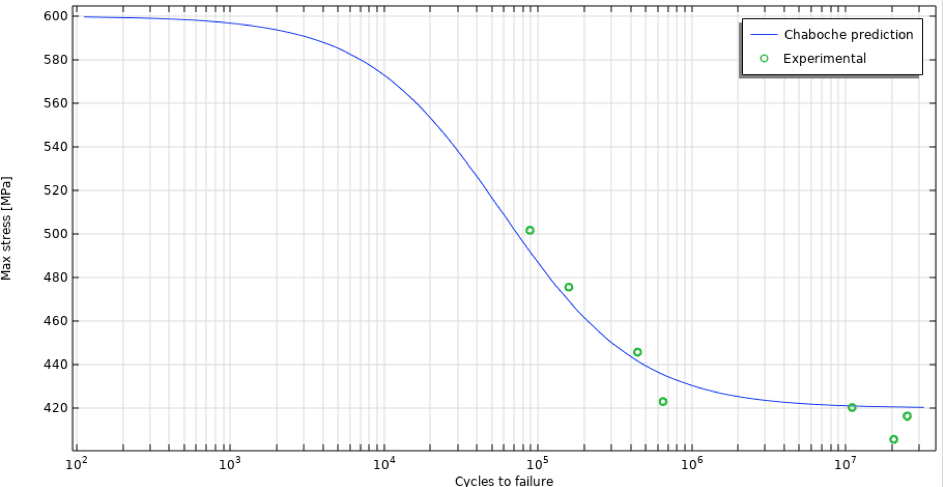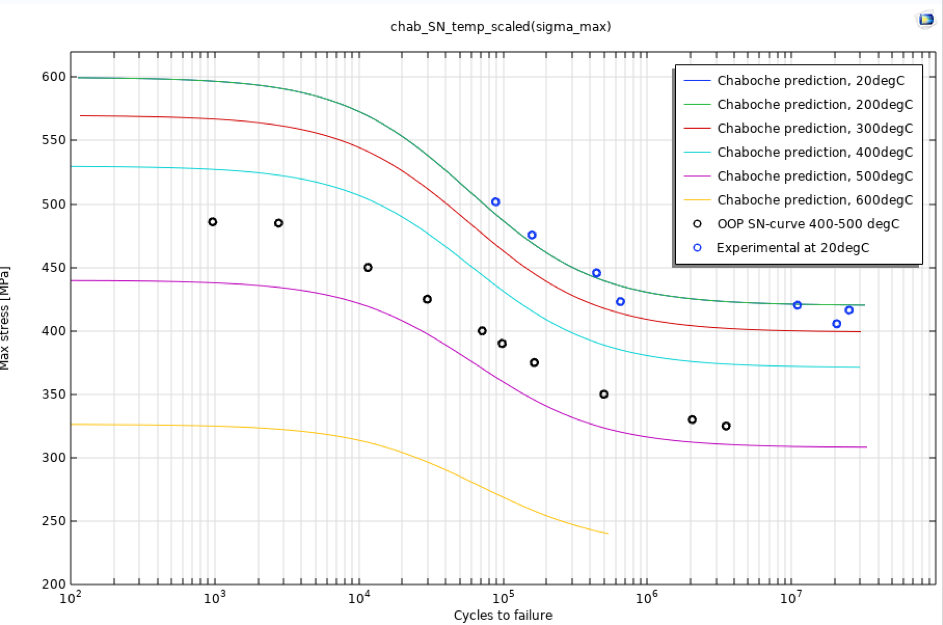### 计算模型

#### 几何模型：实验比较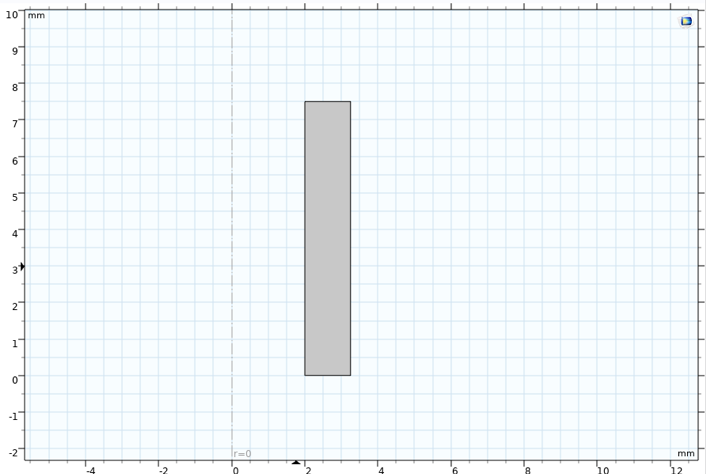#### 几何模型：疲劳评估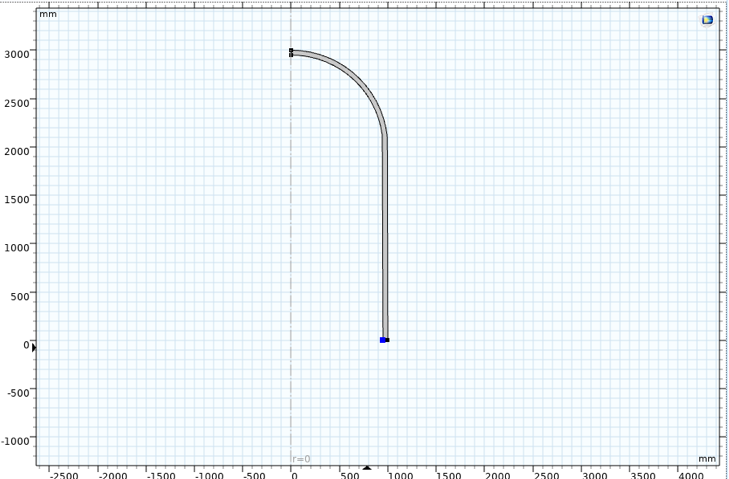#### 网格：实验比较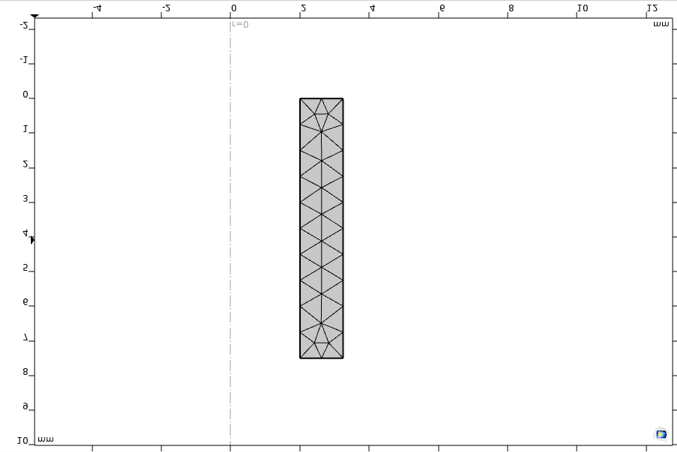#### 网格：疲劳评估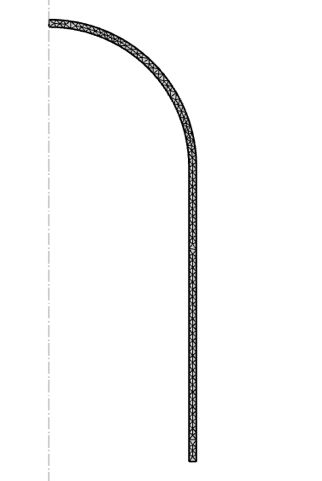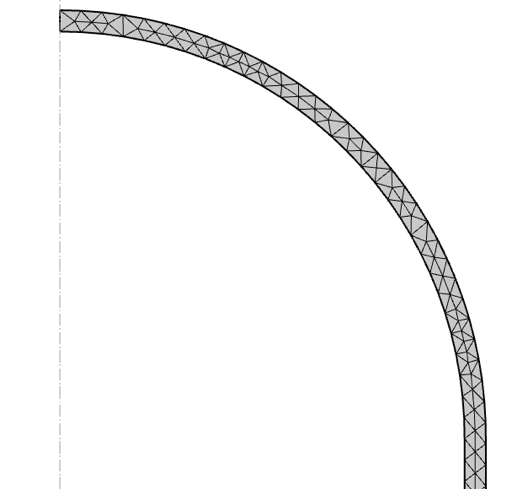#### 物理场设置：材料、载荷和边界条件（实验比较）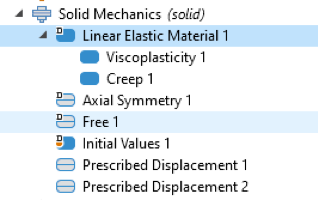### 参数

#### 温度函数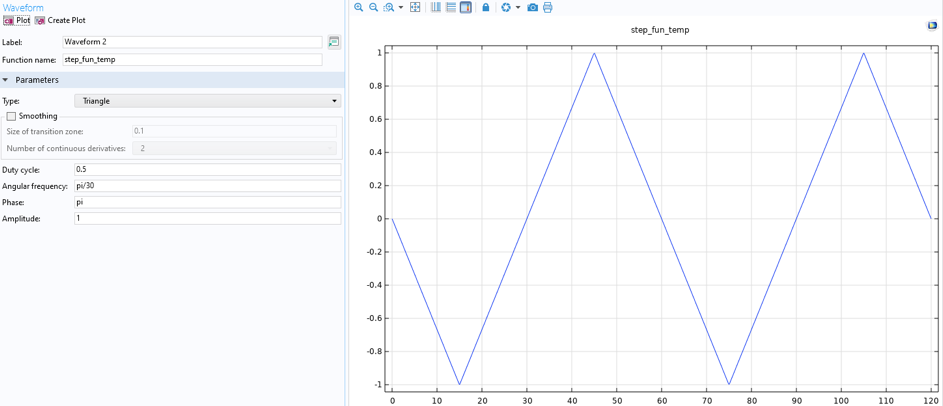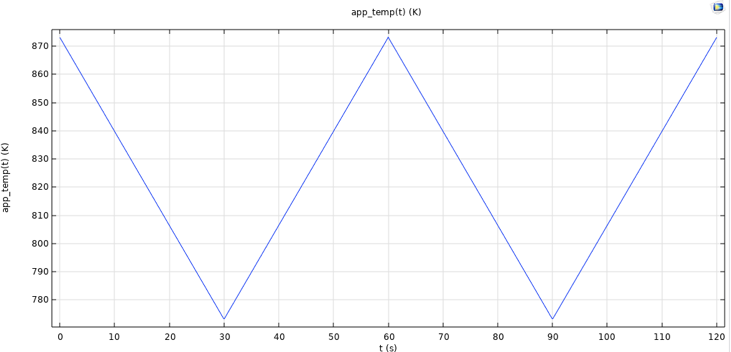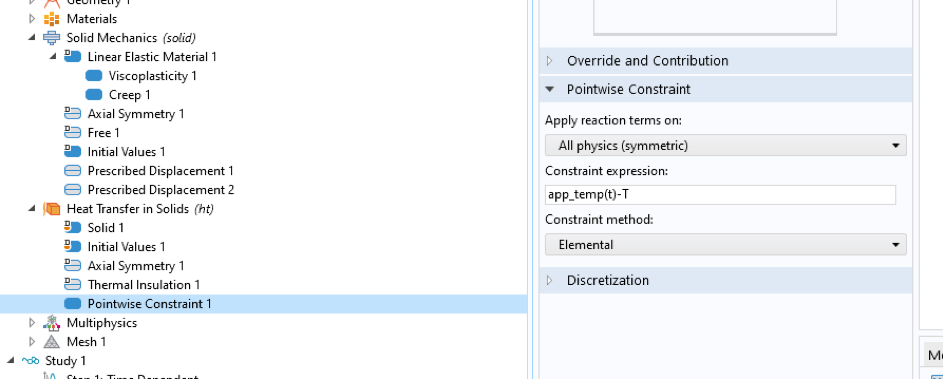#### 载荷函数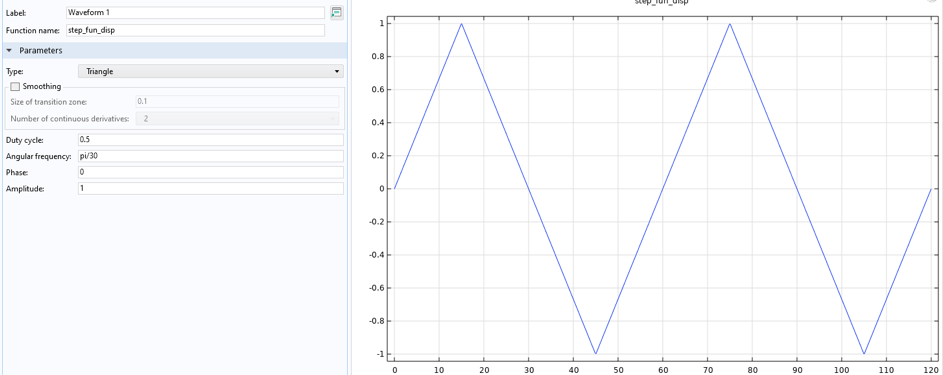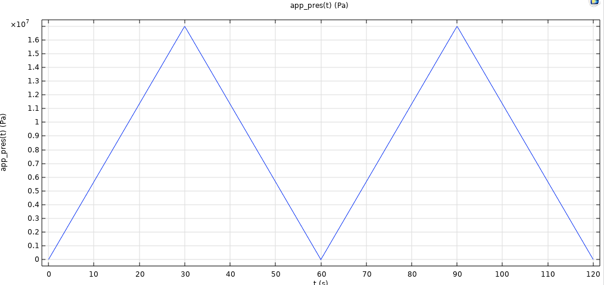#### 材料参数函数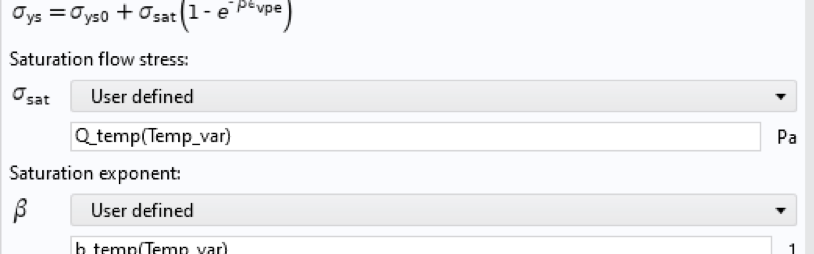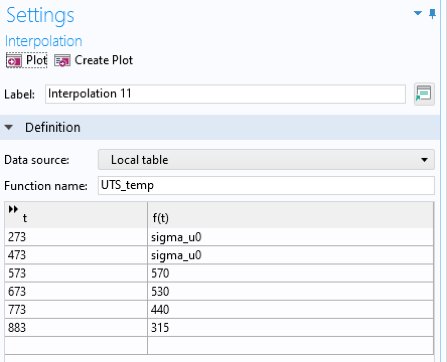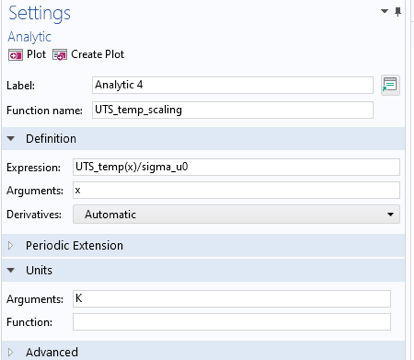### 结果：实验比较

#### 等温测试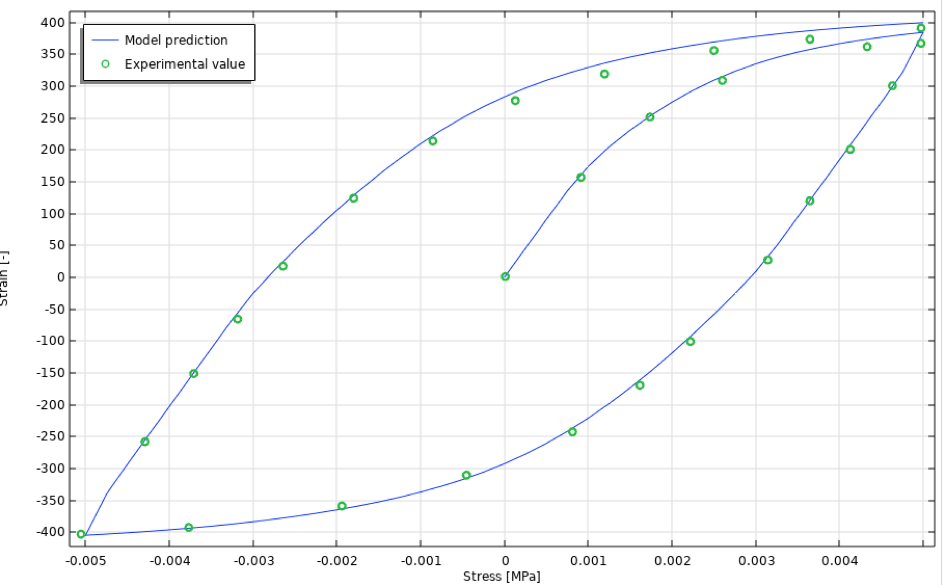19 初始循环应力应变曲线，等温情况。

#### 非等温测试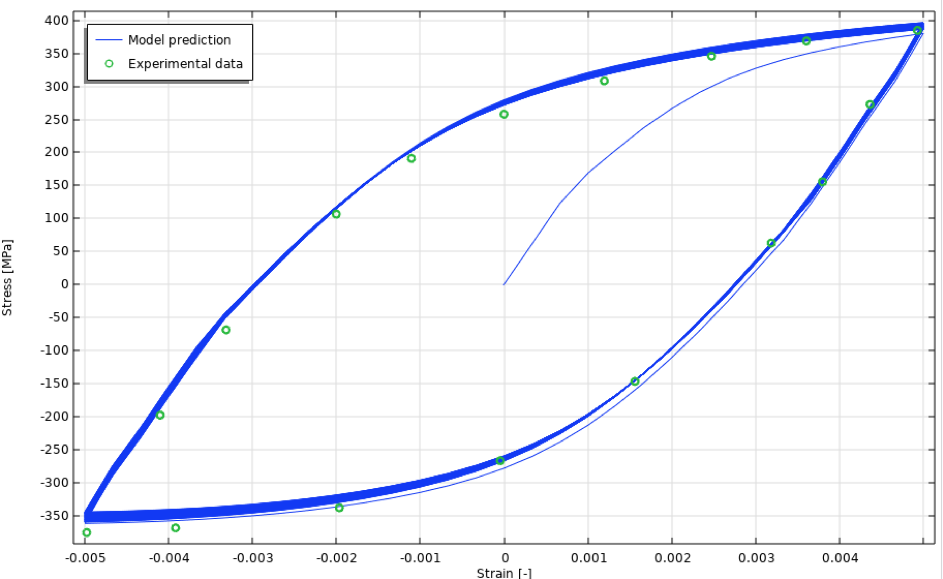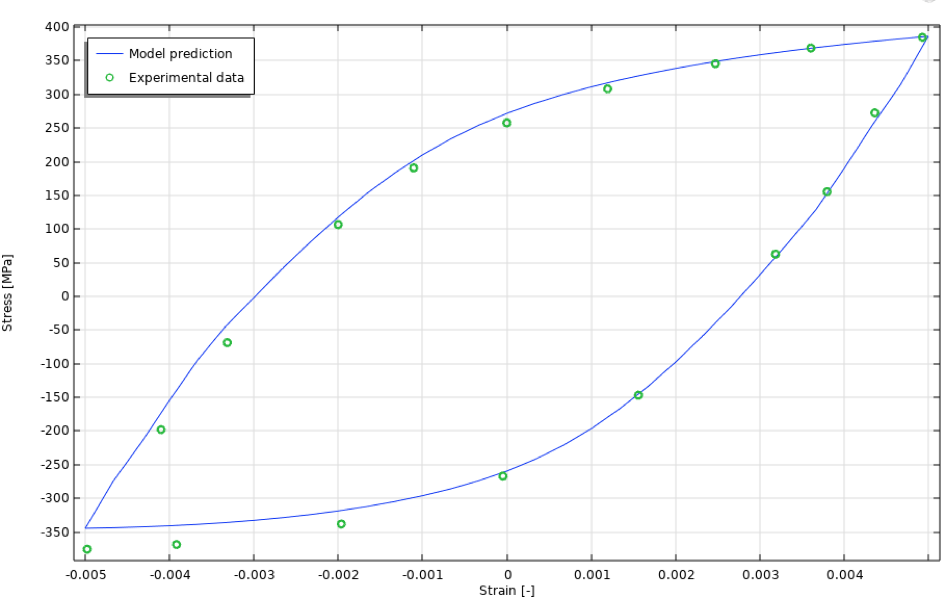21 50次循环应力应变曲线，等温情况。

### 结果：疲劳评估

#### 应力和应变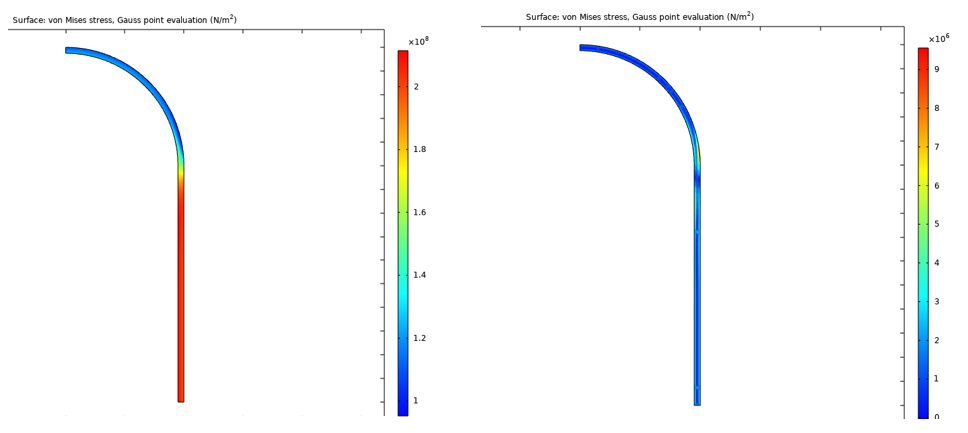50 次循环后的等效黏塑性和蠕变应变如图 23 所示。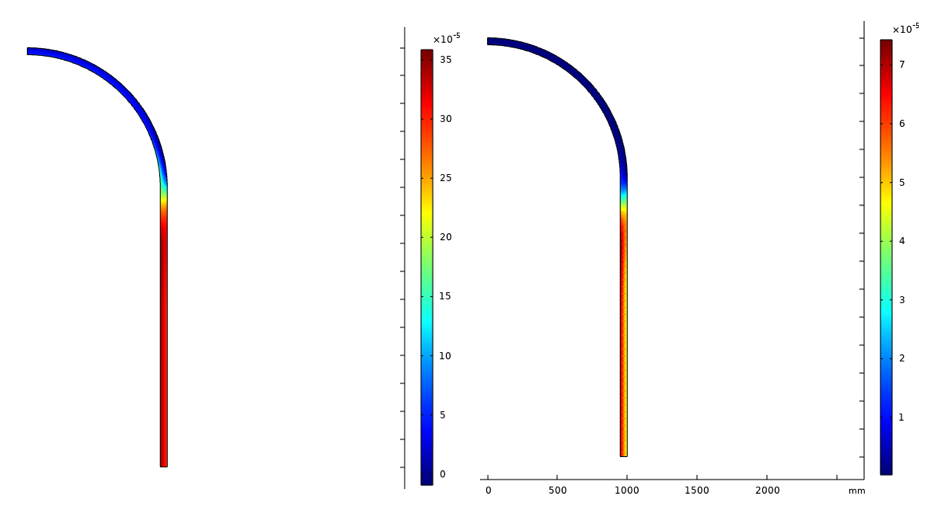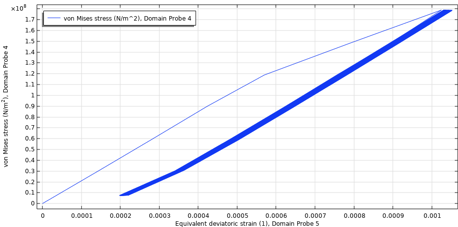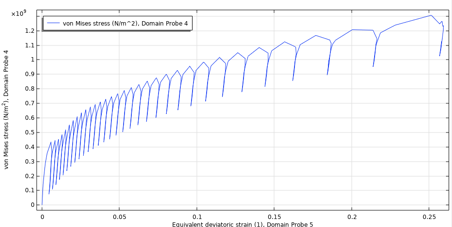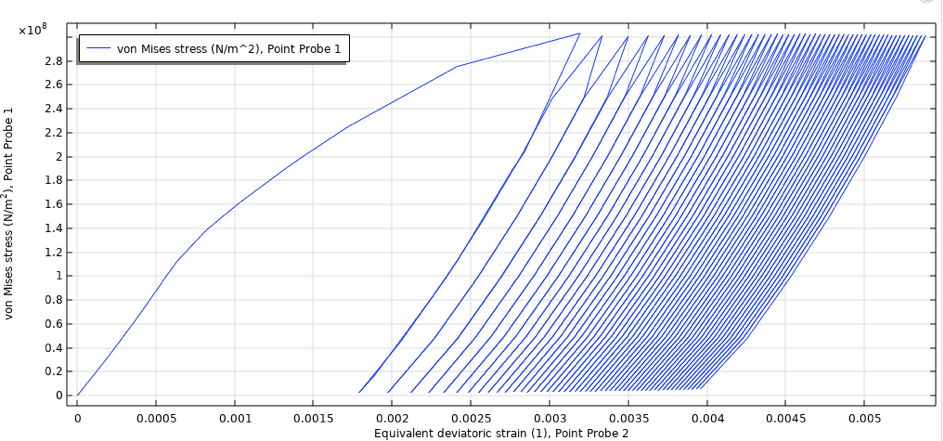#### 计算疲劳周期

\frac{1}{N_F(\sigma_M, \bar\sigma, T^*)}=\frac{1}{\Delta t} \int_{0}^{\Delta t} \frac{dt}{N_F(\sigma_M, \bar\sigma, T(t))}

dD=f(\sigma_M, \bar\sigma, D, T^*)dN

N^*_F=\bigg(\frac{1}{\Delta t} \int_{0}^{\Delta t} \frac{dt}{N_F(\sigma_M, \bar\sigma, T(t))}\bigg)^{-1}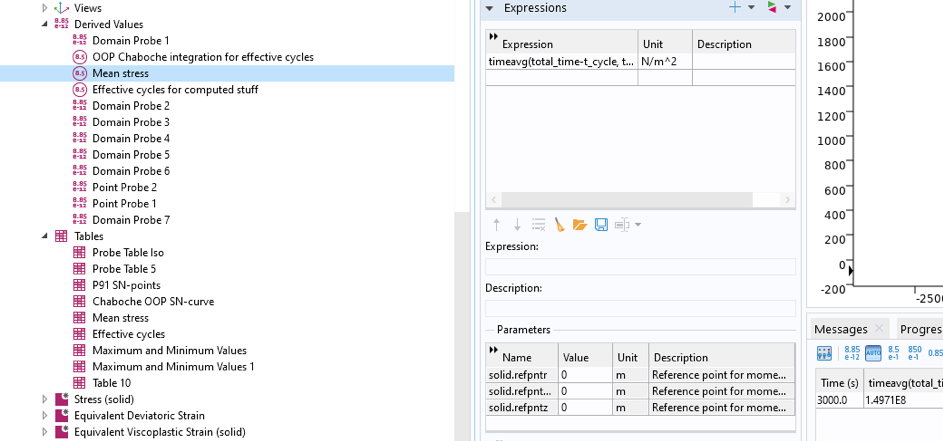26 稳定循环的平均应力的计算。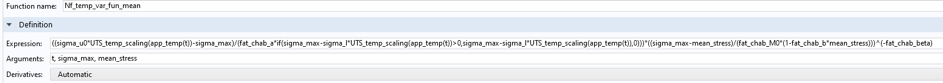27 根据时间、最大应力和平均应力计算的失效循环次数的定义。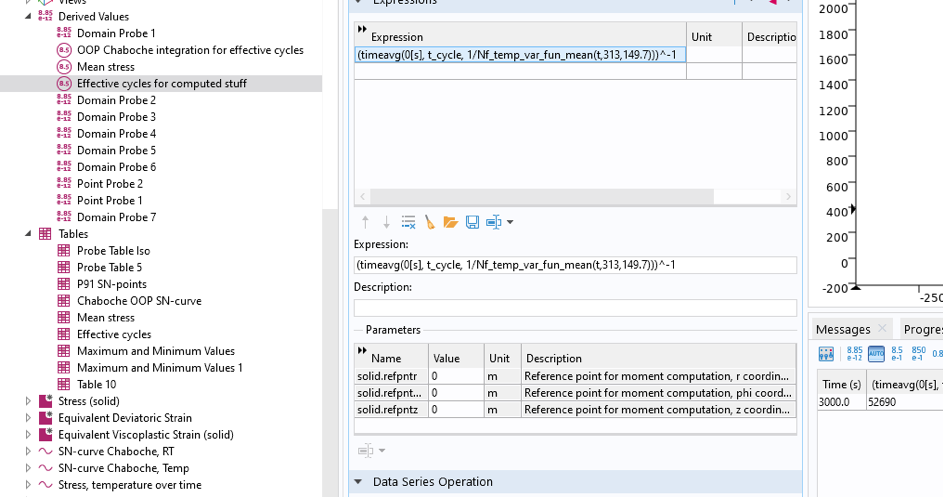28 使用 全局评估 节点计算失效的循环数。

### 结论与讨论

Björn Fallqvist 是Lightness by Design 公司的顾问，负责基于数值分析的产品开发。他于 2016 年在皇家理工学院获得博士学位，致力于开发结构模型以捕获生物细胞的机械行为。他的主要专业兴趣和专业领域是材料表征以及使用各种材料模型捕获物理现象。

1. S. Natesan et al., Preliminary Materials Selection Issues for. s.l. : Argonne National Laboratory, 2006.
2. C.J. Hyde et al., “Thermo-mechanical fatigue testing and simulation using a viscoplasticity model for a P91 steel”, Computational materials science, no. 56, 2012.
3. J.L. Chaboche and P.M. Lesne, “A non-linear continuous fatigue damage model”, Fatigue fracture and Engineering Materials Structures, vol. 11, no. 1, pp. 1&ndash17, 1987.
4. A.A. Saad et al., “Thermal-mechanical fatigue simulation of a P91 steel in a temperature range of 400-600C”, Materials at high temperatures, no. 28, 2011.
5. COMSOL Multiphysics, Structural Mechanics Module User’s Guide.
6. X. Feng et al., “Determination of creep properties of P91 by small punch testing”, Materials at high temperatures, vol. 32, no. 4, 2015.
7. Y. Gorash and D. MacKenzie, Open Eng, vol. 7, 2017.

#### 评论 (6)

##### 留言##### 康 黄
2021-06-23##### hao huang
2021-10-08 COMSOL 员工##### 齐叠 林
2021-07-28##### hao huang
2021-10-08 COMSOL 员工##### pentung wong
2021-09-28##### hao huang
2021-10-08 COMSOL 员工